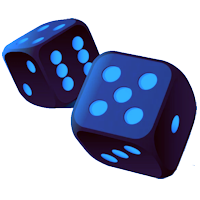Games
Problems
Go Pro!This morning's question is, in essence, if you know the probability of an event happening, what is the probability of it NOT happening?

To answer this question, it's good to remember that a probability is a ratio. It is: [number of desired outcomes] : [total number of outcomes] (assuming that all outcomes are equally likely).

Let's consider the flipping of a coin. Suppose you want the probability of getting heads. The number of desired outcomes is 1. The total number of outcomes is 2.*

'So the probability is 1/2. So what is the probability of NOT getting heads? Well, that's the same as the probability of getting tails. Desired outcomes: 1, possible outcomes 2. Thus, the probability is also 1/2.

* I've had students argue that a coin could land on its rim, so there are actually 3 possible outcomes. I point out to them that there are a couple problems with that. First, if it lands on the rim, we re-roll the coin, so that really doesn't count. Second, if we decided to keep that roll, we can't use our ratio definition above, because all outcomes are not equally likely.

So now let's switch gears and talk about a six-sided die. What is the probability of getting a perfect square when you roll the die? Well, there are 2 perfect squares that are possible results: 1, and 4. So desired outcomes = 2, total outcomes  = 6. Thus the probability is 2/6 (we can simplify that to 1/3, but for now I'd like to keep in the form 2/6).

What is the probability of NOT getting a perfect square? Well, there are 4 numbers that aren't perfect squares: 2, 3, 5, and 6. Desired outcomes = 4, total outcomes = 6. Probability is 4/6.

Let's try one more example. What is the probability of rolling a total of 12 if you roll two six-sided dice?

There is only one way to get a sum of 12: you need a six on each die. There is a total of 6 x 6 = 36 possible outcomes. Desired = 1, Total = 36, probability = 1/36. What about the probability of NOT rolling a 12? Well, you could list off all the possible outcomes, but let's reason this out instead. If there are 36 possible outcomes, and only one of them leads to a sum of 12, how many don't lead to a sum of 12? The answer should be fairly obvious: 36 - 1 = 35. Desired = 35, total = 36, probablity = 35/36.

Now let's put those results together:

Coin: 1/2; 1/2
Die: 2/6; 4/6
2 Dice: 1/36; 35/36

Hopefully you can see a pattern here: in each case, the probabilities add to 1. And, if you think about how we reasoned out the example with two dice, that should make sense to you; every outcome is either a desired or a not desired outcome. There are no other options. So when you add together those options, you get the total number of outcomes. And [total outcomes]/[total outcomes] = 1.

We can write this rule as an equation like this: P(x) + P(~x) = 1.

In words: The probability of x, plus the probability of NOT x equals 1.

I hope that helps!Like us on Facebook to get updates about new resources